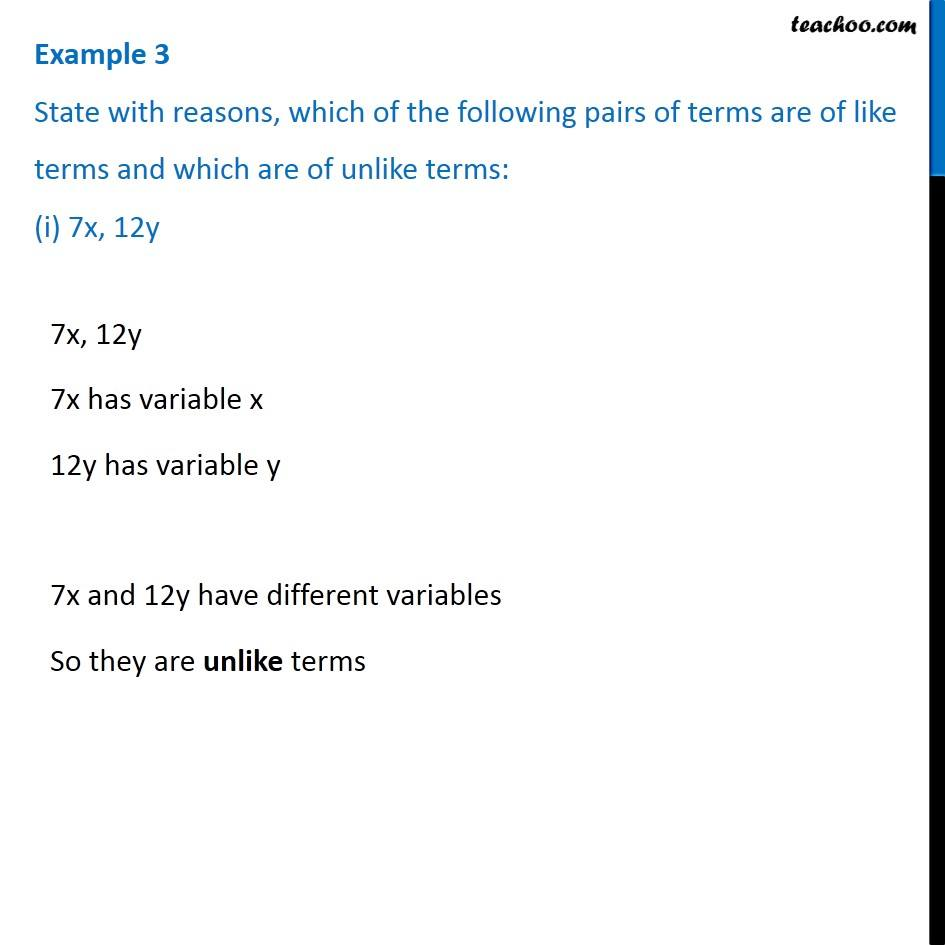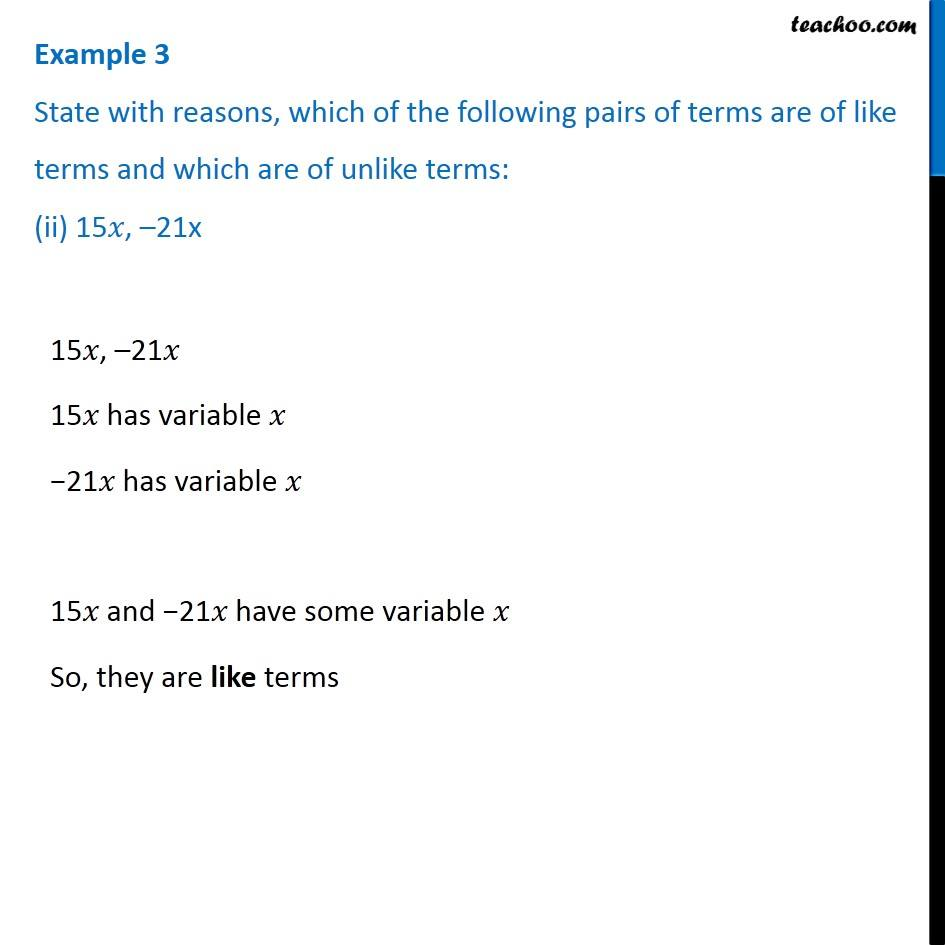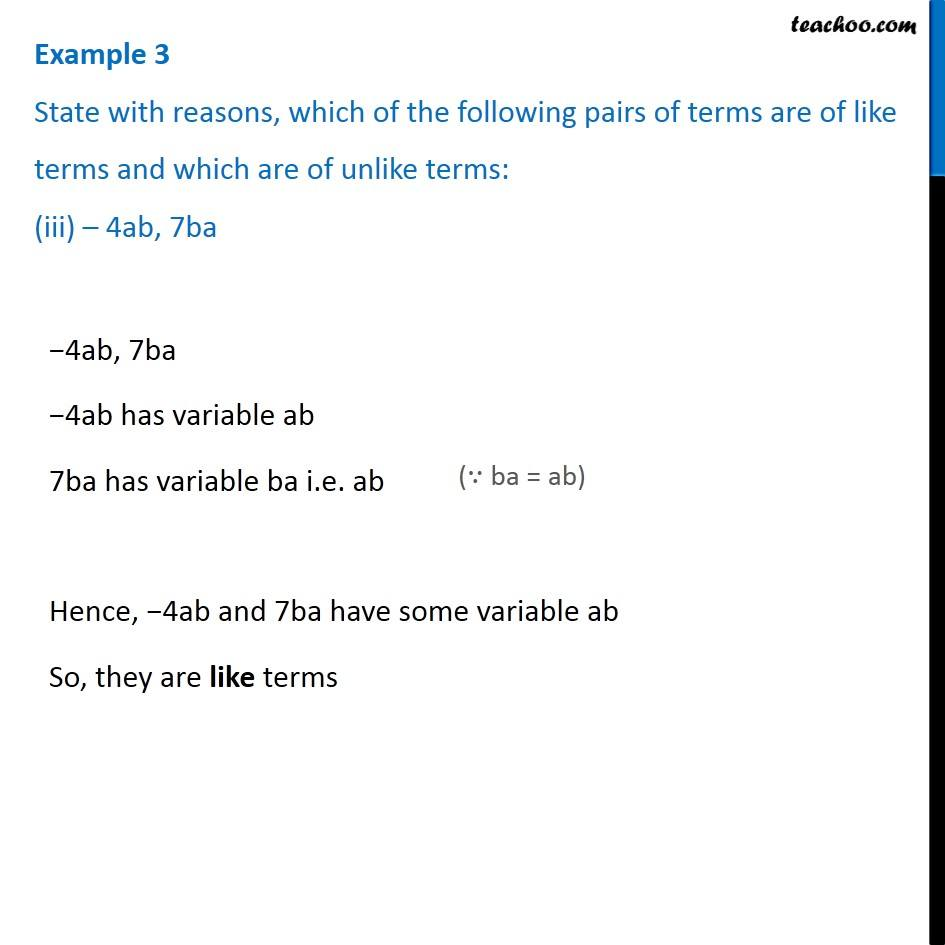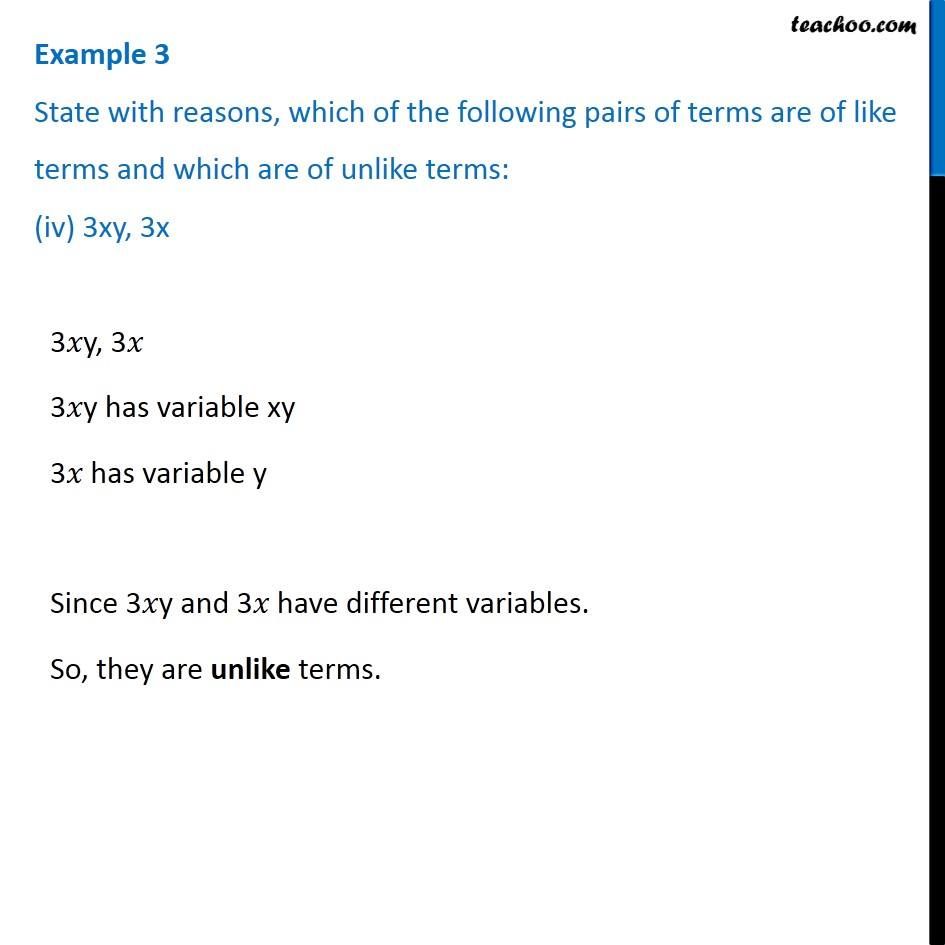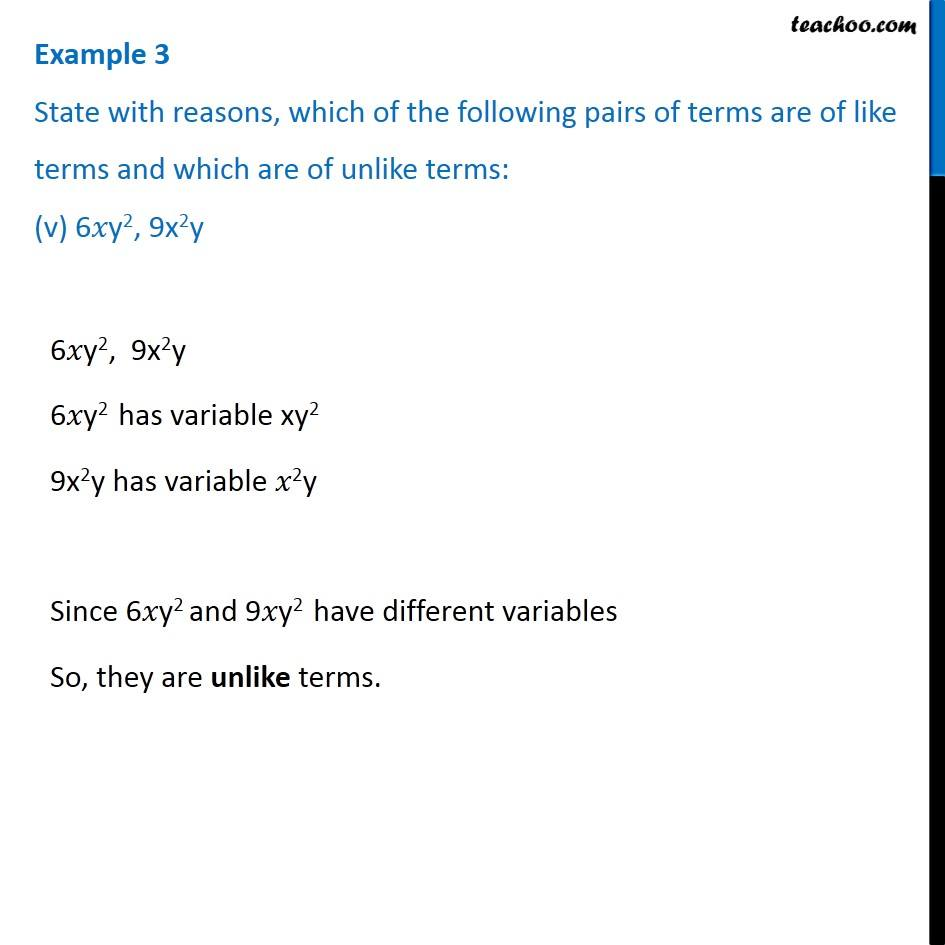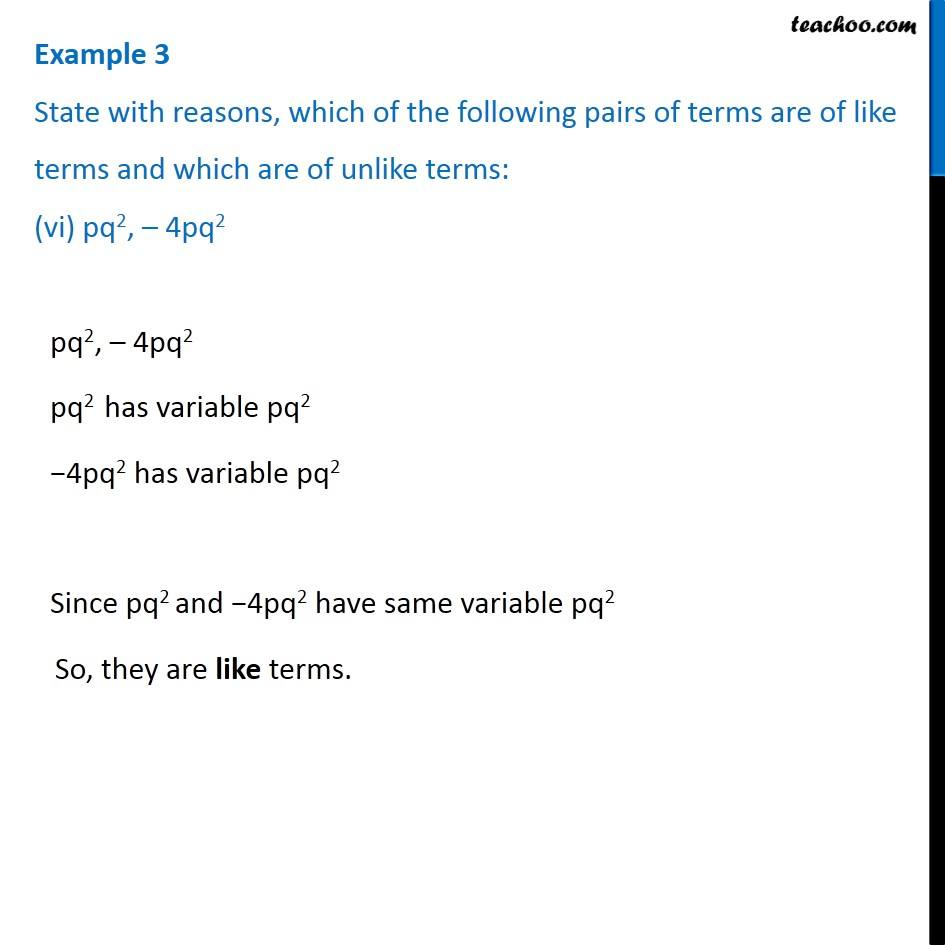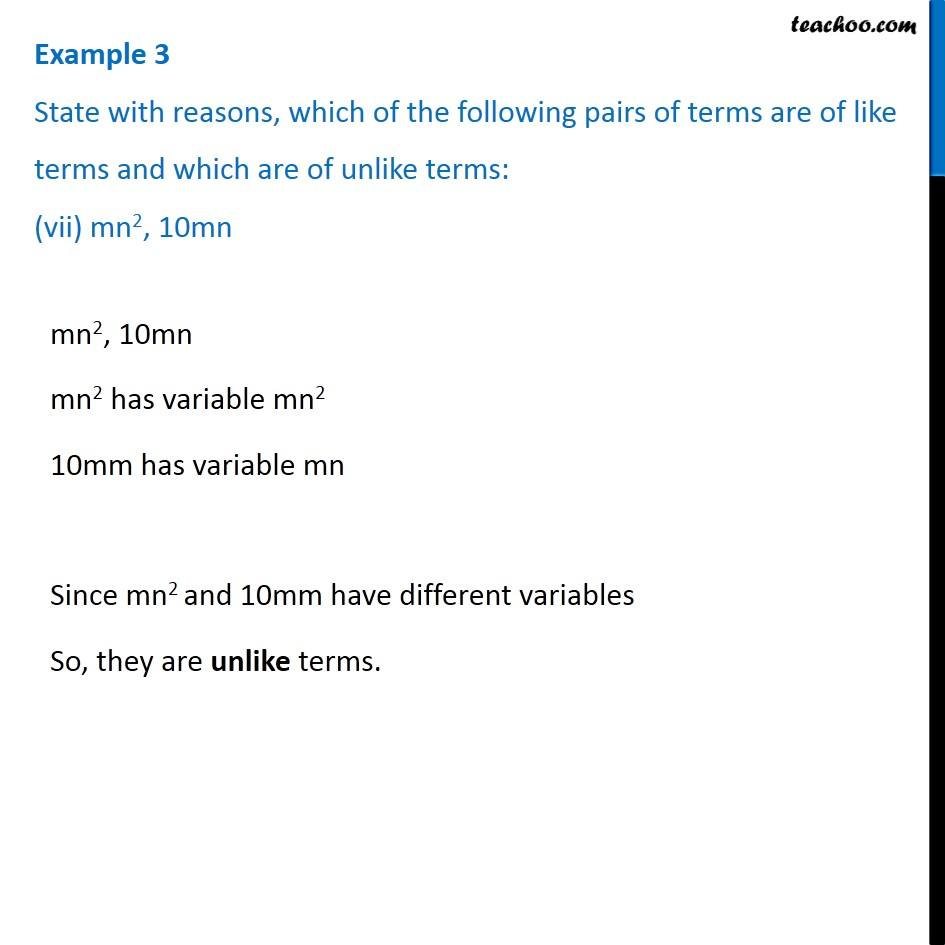1. Chapter 12 Class 7 Algebraic Expressions
2. Serial order wise
3. Examples

Transcript

Example 3 State with reasons, which of the following pairs of terms are of like terms and which are of unlike terms: (i) 7x, 12y 7x, 12y 7x has variable x 12y has variable y 7x and 12y have different variables So they are unlike terms Example 3 State with reasons, which of the following pairs of terms are of like terms and which are of unlike terms: (ii) 15𝑥, –21x 15𝑥, –21𝑥 15𝑥 has variable 𝑥 −21𝑥 has variable 𝑥 15𝑥 and −21𝑥 have some variable 𝑥 So, they are like terms Example 3 State with reasons, which of the following pairs of terms are of like terms and which are of unlike terms: (iii) – 4ab, 7ba −4ab, 7ba −4ab has variable ab 7ba has variable ba i.e. ab (∵ ba = ab) Hence, −4ab and 7ba have some variable ab So, they are like terms Example 3 State with reasons, which of the following pairs of terms are of like terms and which are of unlike terms: (iv) 3xy, 3x 3𝑥y, 3𝑥 3𝑥y has variable xy 3𝑥 has variable y Since 3𝑥y and 3𝑥 have different variables. So, they are unlike terms. Example 3 State with reasons, which of the following pairs of terms are of like terms and which are of unlike terms: (v) 6𝑥y2, 9x2y 6𝑥y2, 9x2y 6𝑥y2 has variable xy2 9x2y has variable 𝑥2y Since 6𝑥y2 and 9𝑥y2 have different variables So, they are unlike terms. Example 3 State with reasons, which of the following pairs of terms are of like terms and which are of unlike terms: (vi) pq2, – 4pq2 pq2, – 4pq2 pq2 has variable pq2 −4pq2 has variable pq2 Since pq2 and −4pq2 have same variable pq2 So, they are like terms. Example 3 State with reasons, which of the following pairs of terms are of like terms and which are of unlike terms: (vii) mn2, 10mn mn2, 10mn mn2 has variable mn2 10mm has variable mn Since mn2 and 10mm have different variables So, they are unlike terms.

Examples